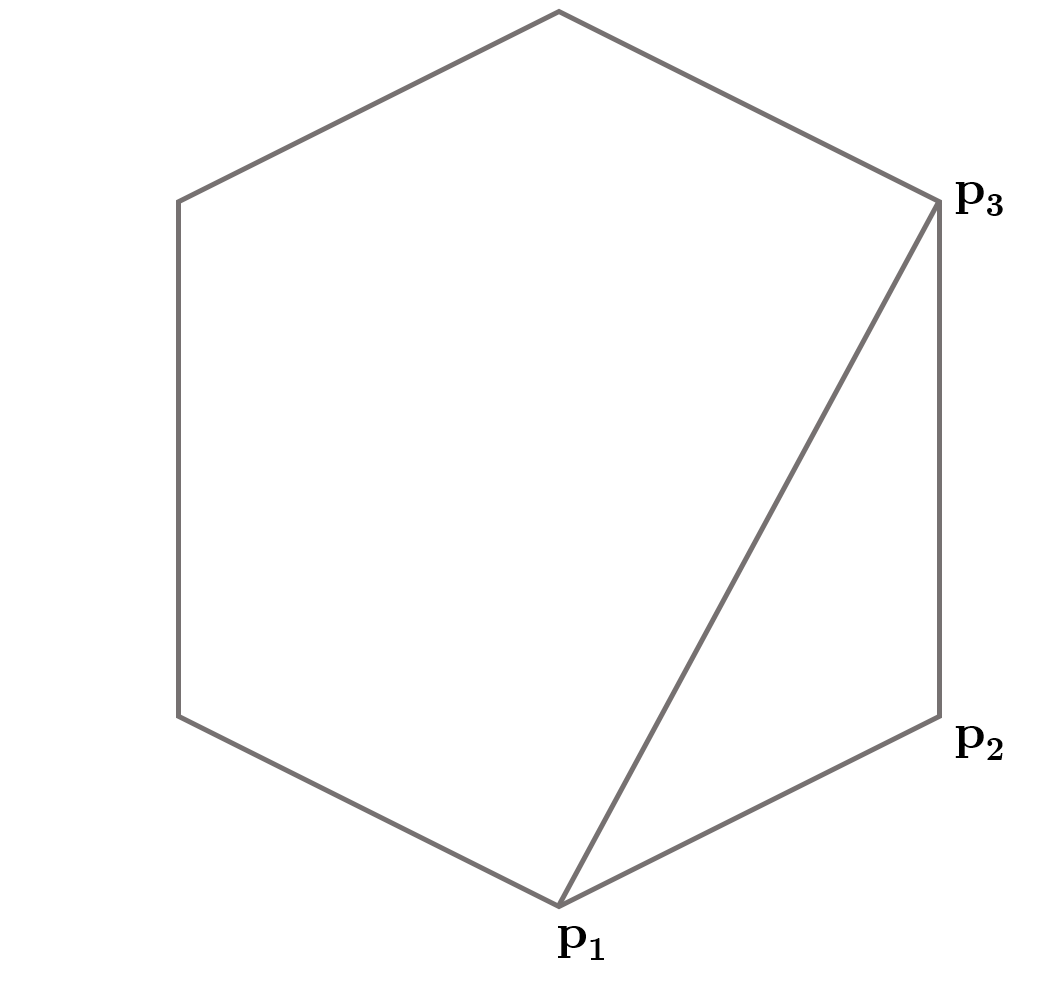You are not logged in. Please login at www.codechef.com to post your questions!

×

# MINPOLY - Editorial

Author: Praveen Dhinwa
Tester: Utkarsh Lath
Editorialist: Balajiganapathi Senthilnathan
Russian Translator: Sergey Kulik
Mandarian Translator: Gedi Zheng

Hard

Geometry

# PROBLEM:

Given $n$ points each with a positive weight, find the minimum weighted convex polygon with $k$ vertices chosen among the $n$ points. Do this for all $k$ from $3$ to $n$. The weight of a polygon is the sum of the weights of the points enclosed by it (including the boundaries).

# SHORT EXPLANATION

Suppose we fix a point $p_1$ as the lower right corner of the polygon we are about to construct. We will sort all the valid points (i.e. those that are above $p_1$) in counter clock wise direction with respect to $p_1$. Now let us define $dp(p_3, p_2, k)$ as the minimum weighted polygon which has $p_1$ as the lowest point, $p_3$ and $p_2$ are the last two added points and we have to add $k$ more vertices. Now, for all vertices in counter clock wise order, we try adding each point $i$ which is to the left of the line connecting $p2$ to $p3$ and recursively solve the $dp(i, p3, k + 1)$ and add we also add the weights of the point inside the triangle $p2 - p3 - i$. We take minimum over all such $i$ and that will be the answer for $dp(p_3, p_2, k)$.

Now we try fixing each of the point as the lower most one (i.e. $p_1$) and do the above procedure. For each $k$, we take minimum over all such fixed points. We do this for all $k$ (3 \leq k \leq n) to get the final answer$. # EXPLANATION: Let us fix a point$p_1$as the bottom most point of the polygon (i.e. the one with the least$y$coordinate). Since this is the bottom most point, we can ignore all the points which have$y$values less than$p_1$. Now we sort the remaining points in counter clockwise direction. We want to get the minimum weighted convex polygon for each$k$having$p_1$as the bottom most point.The key observation is that suppose we have a minimum weighted convex polygon with$k$vertices, then if we remove any vertex, we will be left with a minimum weighted convex polygon of$k - 1$vertices having$p_1$as the bottom most point. So, to get the answer for$k$, we try adding each valid point as the next vertex and solve the problem for$k - 1$vertices. Then take the minimum over all such valid points to get the minimum value for$k$vertices. In other words, we are building the polygon triangle by triangle. What other information are needed for implementing this? 1. We need to know the last two added points added to the polygon. This is to ensure that the next point we add does make a convex polygon (i.e it has to be to the left of the line made by the last two points). 2. We need to know the weight of all possible triangles. We can pre-calculate this in$O(n^4)$time and$O(n^3)$space. Let us define$totalWeightsInside[i][j][k]$as the weight of the points inside the triangle formed by points$i, j$and$k$. We can calculate this by looping through all points and adding its weight to$totalWeightsInside[i][j][k]$if that point lies inside the triangle formed by$i, j$and$k$. Let the function$dp(p_3, p_2, k)$return the minimum weighted convex polygon with$p_1$as the bottom most vertex,$p_3$and$p_2$as the last two vertex and$k$vertices remaining to be added. Now we loop through all valid points$i$which are ahead of$p_3$in counter clockwise order and see if can add$i$to the polygon. We can add$i$only if it is to the left of the line formed by$p_2 - p_3$. Otherwise the angle made by$p_2 - p_3 - i$will be greater than 180 degrees and hence it won't be a convex polygon. Now to get the weight, we recursively solve for$dp(i, p3, k - 1)$and add the weights of the points inside the triangle formed by$p_2 - p_3 - i$to the weight. We try this for all$i$and the minimum among them would be the answer. Finally note that for a fixed$p_1$, the state may repeat. So, we memoize the values to avoid recalculation. For getting the final answer, we loop through all points and fix them as$p_1$. Then we store all the points which are not below$p_1$and sort them in counter clockwise order. Now we have to select the next 2 vertices of the polygon. So we loop through all pairs of valid points and keep them as the next 2 vertices. Finally we need to solve for all$k$, so we will loop for$k$from 3 to$n$and call$dp(p3, p2, k - 3)$to get the answer for this case. We keep updating the minimum weighted polygon for$k$vertices using this value. Please consult author's solution for the sample implementation of this idea. ## Time Complexity:$\mathcal{O} (n^5)$•$\mathcal{O}(n)$for fixing vertex$p_1$•$dp(p_3, p_2, k)$has total number of states equal to$\mathcal{O}(n^3)$and total number of transitions equal to$\mathcal{O}(n)$. Hence, time taken will be$\mathcal{O}(n^4)$. • Finally, the total time will be product of both, i.e.$\mathcal{O}(n^5)$# AUTHOR'S AND TESTER'S SOLUTIONS: This question is marked "community wiki". asked 22 May '15, 21:0175542742 accept rate: 77%0★admin ♦♦ 19.8k350498541  toggle preview community wiki: Preview ### Follow this question By Email: Once you sign in you will be able to subscribe for any updates here By RSS: Answers Answers and Comments Markdown Basics • *italic* or _italic_ • **bold** or __bold__ • link:[text](http://url.com/ "title") • image?![alt text](/path/img.jpg "title") • numbered list: 1. Foo 2. Bar • to add a line break simply add two spaces to where you would like the new line to be. • basic HTML tags are also supported • mathemetical formulas in Latex between$ symbol

Question tags:

×15,852
×1,359
×647
×74
×20

question asked: 22 May '15, 21:01

question was seen: 2,173 times

last updated: 11 Feb '16, 17:36